# Test: Sets- 2

## 30 Questions MCQ Test GATE Computer Science Engineering(CSE) 2023 Mock Test Series | Test: Sets- 2

Description
Attempt Test: Sets- 2 | 30 questions in 90 minutes | Mock test for Computer Science Engineering (CSE) preparation | Free important questions MCQ to study GATE Computer Science Engineering(CSE) 2023 Mock Test Series for Computer Science Engineering (CSE) Exam | Download free PDF with solutions
QUESTION: 1

### The following is the incomplete operation table a 4-element group.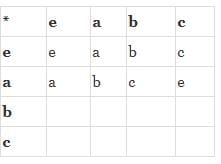Q.  The last row of the table is

Solution:

It is given that the given set of 4 elements is group. The element 'e' is clearly identity as the row corresponding to it has all values same as the other operand. Also, since a*c is e, c*a should also be e which is only the case in option D

QUESTION: 2

### The inclusion of which of the following sets into S = {{1, 2}, {1, 2, 3}, {1, 3, 5}, (1, 2, 4), (1, 2, 3, 4, 5}} is necessary and sufficient to make S a complete lattice under the partial order defined by set containment ?

Solution:
• A partially ordered set L is called a complete lattice if every subset M of L has a least upper bound called as supremum and a greatest lower bound called as infimum.
• We are given a set containment relation.
• So, supremum element is union of all the subset and infimum element is intersection of all the subset.
• Set S is not complete lattice because although it has a supremum for every subset, but some subsets have no infimum. We take subset {{1,3,5},{1,2,4}}.Intersection of these sets is {1}, which is not present in S. So we have to add set {1} in S to make it a complete lattice

Thus, option (A) is correct. Please comment below if you find anything wrong in the above post.

QUESTION: 3

### How many graphs on n labeled vertices exist which have at least (n2 - 3n)/2 edges ?

Solution:

Let there be total number of edges of a graph to be formed be e and total number of vertices be n.

So the total number of ways a graph can be formed having exactly e edges and v vertices is given by the total number of ways we can select e edges among v edges

i. e.

C (v, e)

Now, here e = (n2 – 3n)/2 and v = n(n-1)/2 (maximum number of edges in a simple graph).

So to select a edge we can do that in C (v, (n2 – 3n)/2) ways.

Since minimum no. of edges to be selected for a graph are (n2 – 3n)/2.

So total number of graphs possible will be :

C(v, e) + C(v, e+1) + C(v, e+2) +…………...+ C(v, v).

C(v, v-e) + C(v, v-(e+1)) + C(v, v-(e+2)) +…………...+ C(v, v-v).

Since v - e = n(n-1)/2 - (n2 – 3n)/2 = n

Therefore

C(v, n) + C(v, n-1) + C(v, n-2) +…………...+ C(v, 0).

Solving this we will get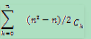QUESTION: 4

Consider the set ∑* of all strings over the alphabet ∑ = {0, 1}. ∑* with the concatenation operator for strings

Solution:
QUESTION: 5

Let (5, ≤) be a partial order with two minimal elements a and b, and a maximum element c.

Let P : S → {True, False} be a predicate defined on S.
Suppose that P(a) = True, P(b) = False and P(x) ⇒ P(y) for all x, y ∈ S satisfying x ≤ y, where ⇒ stands for logical implication.

Q. Which of the following statements CANNOT be true ?

Solution:

'a' and 'b' are given as minimal elements. No other element in S is of lower order than either a or b. 'c' is given as maximum element. So, c is of higher order than any other element in S.
P(a) = True means all elements 'x' which have an edge from element 'a' have to be true. Since there is an edge from 'a', we have to satisfy formula P(a) => P(x), which can only be done by setting P(x) = True.
Elements which have an edge from b can be anything because formula P(b) => P(x) is satisfied as P(b) = False.
(A) This statement is true because making all elements true trivially satisfy formula P(x) => P(y).
(B) This statement is true if all elements are connected from b then all elements can be false.
(C) This statement is true because b<=x ensures x!=a and for all other elements P(x) can be false without violating the given implication.
(D) This statement is false. Since, P(a) = true , for all 'x' such that a<=x, P(x) must be true. We do have at least one such 'x', which is 'c' as it is the maximum element.

Thus, option (D) is the answer.

Please comment below if you find anything wrong in the above post.

QUESTION: 6

Let f : A → B be an injective (one-to-one) function.

Define g : 2A → 2B as :
g(C) = {f(x) | x ∈ C}, for all subsets C of A.
Define h : 2B → 2A as :
h(D) = {x | x ∈ A, f(x) ∈ D}, for all subsets D of B.

Q.
Which of the following statements is always true ?

Solution:
QUESTION: 7

Let ∑ = (a, b, c, d, e) be an alphabet. We define an encoding scheme as follows : g(a) = 3, g(b) = 5, g(c) = 7, g(d) = 9, g(e) = 11.

Let pi denote the i-th prime number (p1=2)
For a non-empty string s=a1…an, where each a∈ ∑ define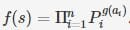For a non-empty sequence (sj, ........ sn) of string from  ∑+ defines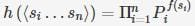Q.
Which of the following numbers is the encoding h of a non-empty sequence of strings ?

Solution:

Since, the answer is a product of three prime numbers 2, 3 and 5. So, we have three non-empty sequence of strings : "a", "a" and "a".
f(s) = 2x for some x Since, 7 and 9 are not multiple of 2. So, options (A) and (C) are eliminated.
f(a) = 23 = 8 h = 28 3858

Thus, option (B) is correct.

Please comment below if you find anything wrong in the above post.

QUESTION: 8

Which of the following is true?

Solution:

A group is a set of elements such that any two elements of the group combine to form a third element of the same group. Also, a group must satisfy certain properties: Closure Property - Any two elements of the set when operated open by an operator form a third element that must also be in the set. Associative Property - For an expression with three or more operands having the same operator between them, the order of operation does not matter as long as the sequence of operands are not changed. For example, (a + b) + c = a + (b + c). Identity element Property - Each set must have an identity element, which is an element of the set such that when operated upon with another element of the set, it gives the element itself. For example, a + 0 = a. Here, 0 is the identity element. Invertibility Property - For each element of the set, inverse should exist.   Now, for the given statements, we have A is incorrect as it does not satisfies closure property. If we take two negative numbers and multiply them, we get a positive number which is not in the set. B is correct. The matrices in the set must be non - singular, i.e., their determinant should not be zero, for the inverse to exist (Invertibility Property). C is incorrect as the inverse of a singular (determinant = 0) matrix does not exist (Invertibility Property violated). Thus, B is the correct option. Please comment below if you find anything wrong in the above post.

QUESTION: 9

The binary relation S = ф (empty set) on set A = {1, 2, 3} is :

Solution:
• Reflexive : A relation is reflexive if every element of set is paired with itself. Here none of the element of A is paired with themselves, so S is not reflexive.
• Symmetric : This property says that if there is a pair (a, b) in S, then there must be a pair (b, a) in S. Since there is no pair here in S, this is trivially true, so S is symmetric.
• Transitive : This says that if there are pairs (a, b) and (b, c) in S, then there must be pair (a,c) in S. Again, this condition is trivially true, so S is transitive. Thus, option (D) is correct. Please comment below if you find anything wrong in the above post.
QUESTION: 10

Consider the following relations

R1(a,b) iff (a+b) is even over the set of integers
R2(a,b) iff (a+b) is odd over the set of integers
R3(a,b) iff a.b > 0 over the set of non-zero rational numbers
R4(a,b) iff |a - b| <= 2 over the set of natural numbers

Q. Which of the following statements is correct?

Solution:

So basically, we have to tell whether these relations are equivalence or not.

1. R1(a,b)
• Reflexive : Yes, because (a+a) is even.
• Symmetrix : Yes, (a+b) is even ⟹ (b+a) is even.
• Transitive : Yes, because (a+b) is even and (b+c) is even ⟹ (a+c) is even.

So R1 is equivalence relation.

2. R2(a,b)

• Reflexive : No, because (a+a) is even.

So R2 is not equivalence relation.

3. R3(a,b)

• Reflexive : Yes, because a.a > 0.
• Symmetrix : Yes, a.b > 0 ⟹ b.a > 0.
• Transitive : Yes, because a.b > 0 and b.c > 0 ⟹ a.c > 0.

So R3 is equivalence relation.

4. R4(a,b)

• Reflexive : Yes, because |a-a| ≤ 2.
• Symmetrix : Yes, |a-b| ≤ 2 ⟹ |b-a| ≤ 2.
• Transitive : No, because |a-b| ≤ 2 and |b-c| ≤ 2 ⇏ (a-c) is even.

So R4 is not equivalence relation.

QUESTION: 11

Consider the following statements:

S1: There exists infinite sets A, B, C such that A ∩ (B ∪ C) is finite.
S2: There exists two irrational numbers x and y such that (x+y) is rational.

Q. Which of the following is true about S1 and S2?

Solution:

S1: A ∩ (B ∪ C) Here S1 is finite where A, B, C are infinite We’ll prove this by taking an example. Let A = {Set of all even numbers} = {2, 4, 6, 8, 10...} Let B = {Set of all odd numbers} = {1, 3, 5, 7...........} Let C = {Set of all prime numbers} = {2, 3, 5, 7, 11, 13......} B U C = {1, 2, 3, 5, 7, 9, 11, 13......} A ∩ (B ∪ C) Will be equals to: {2} which is finite. I.e. using A, B, C as infinite sets the statement S1 is finite. So, statement S1 is correct. S2: There exists two irrational numbers x, y such that (x+y) is rational To prove this statement as correct, we take an example. Let X = 2-Sqrt (3), Y = 2+Sqrt (3) => X, Y are irrational X+Y = 2+Sqrt (3) + 2-Sqrt (3) = 2+2 = 4 So, statement S2 is also correct. Answer is Option C Both Statements S1, S2 are correct.

QUESTION: 12

A relation R is defined on the set of integers as xRy if f(x + y) is even. Which of the following state­ments is true?

Solution:

There are 2 2 equivalence classes. 1) All odd integers. (Reflexive as sum of two even is even, Symmetric and Transitive as + operation is Symmetric and Transitive) 2) All Even Integers. (Reflexive as sum of two odd is even, Symmetric and Transitive as + operation is Symmetric and Transitive)

QUESTION: 13

Let P(S) denotes the power set of set S. Which of the following is always true?

Solution:

Ex:  let a set ‘s’ be, s={1,2} Power-set(s)=P(s)={{},{1},{2},{1,2}} Note: ‘{}’ denotes empty or NULL set or φ. In general, if a set ‘s’ contains ‘n’ elements,  power-set will contain 2^n elements, that denotes set of all subset of ‘s’. Solution: Option (a): P(P(S))=P(S) If, S={1} P(S)={ φ, {1}} P(P(S))={ φ,{ φ},{1},{ φ, {1}}} NOTE: LHS is set of set of subsets of ‘S’ and RHS is set of subsets of ‘S’. So, we can conclude this option is false. Option (b): P(P(S)) ∩P(S)={ φ}. If, S={1} P(S)={ φ, {1}} P(P(S))={ φ,{ φ},{1},{ φ, {1}}} P(P(S)) ∩P(S)= { φ}=RHS So, we can conclude this option is TRUE. Option (a): P(S) ∩S=P(S) If, S={1} P(S)={ φ, {1}} P(S) ∩S= φ NOTE: We can’t find anything common in set of elements (S) and set of sets(P(S)). So, we can conclude this option is false. Option (a): S ∉ P(S) If, S={1} P(S)={ φ, {1}} Clearly, S is subset of P(S). NOTE: By definition only, its clear that power-set is set of all subset that will contain S also. So, we can conclude this option is false.

QUESTION: 14

The binary operator ≠ is defined by the following truth table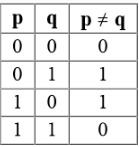Q.  Which one of the following is true about the binary operator ≠?

Solution:

The operation is basically XOR which is both commutative and associative.

QUESTION: 15

Suppose L = {p, q, r, s, t} is a lattice represented by the following Hasse diagram: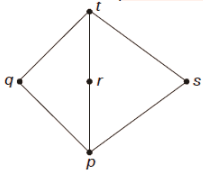For any x, y ∈ L, not necessarily distinct, x ∨ y and x ∧ y are join and meet of x, y respectively. Let L3 = {(x,y,z): x, y, z ∈ L} be the set of all ordered triplets of the elements of L. Let pr be the probability that an element (x,y,z) ∈ L3 chosen equiprobably satisfies x ∨ (y ∧ z) = (x ∨ y) ∧ (x ∨ z). Then

Solution:

Number of triplets in L3 = Number of ways in which we can choose 3 elements from 5 with repetition = 5 * 5 * 5 = 125.

Now, when we take x = t, then the given condition for L is satisfied for any y and z. Here, y and z can be taken in 5 * 5 = 25 ways.

Take x = r, y = p, z = p. Here also, the given condition is satisfied. So, pr > 25 / 125 > 1/5.

For x = q, y = r, z = s, the given condition is not satisfied as q ⋁ (r ⋀ s) = q ⋁ p = q, while (q ⋁ r) ⋀ (q ⋁ s) = t ⋀ t = t.

So, pr ≠ 1.

Hence D choice.

QUESTION: 16

Let R be the relation on the set of positive integers such that aRb if and only if a and b are distinct and have a common divisor other than 1. Which one of the following statements about R is True?

Solution:

R cannot be reflexive as 'a' and 'b' have to be distinct in aRb. R is symmetric if a and b have a common divisor, then b and a also have. R is not transitive as aRb and bRc doesn't mean aRc. For example 3 and 15 have common divisor, 15 and 5 have common divisor, but 3 and 5 don't have.

QUESTION: 17

The cardinality of the power set of {0, 1, 2 . . ., 10} is _________.

Solution:

The power set has 2n elements. For n = 11, size of power set is 2048.

QUESTION: 18

Consider two relations R1(A, B) with the tuples (1, 5), (3, 7) and R1(A, C) = (1, 7), (4, 9). Assume that R(A,B,C) is the full natural outer join of R1 and R2. Consider the following tuples of the form (A,B,C)

a = (1, 5, null),
b = (1, null, 7),
c = (3, null, 9),
d = (4, 7, null),
e = (1, 5, 7),
f = (3, 7, null),
g = (4, null, 9).

Q. Which one of the following statements is correct?

Solution: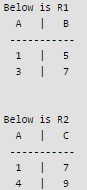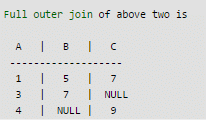So the full outer join contains e = (1, 5, 7), f = (3, 7, null), g = (4, null, 9).

QUESTION: 19

The number of onto functions (surjective functions) from set X = {1, 2, 3, 4} to set Y = {a, b, c} is ________________

Solution:

A function f from X to Y is called onto if for all 'y' in Y there is an 'x' in X such that f(x) = y.          In onto functions, all elements in Y are used. Source: http://www.regentsprep.org/regents/math/algtrig/atp5/OntoFunctions.htm Every Surjective or Onto function sends two elements of {1, 2, 3, 4} to the same element of {a, b, c}. There are 4C2 = 6 such pairs of elements. The pairs are {1, 2}, {1, 3}, {1, 4}, {2, 3}, {2, 4}, {3, 4}. For a given pair {i, j} ⊂ {1, 2, 3, 4}, there are 3! sujective functions such that f(i) = f(j). Hence there are total 6 * 6 = 36 surjective functions.

QUESTION: 20

Let X and Y denote the sets containing 2 and 20 distinct objects respectively and F denote the set of all possible functions defined from X and Y. Let f be randomly chosen from F. The probability of f being one-to-one is _________

Solution:

X has 2 elements Y has 20 elements Number of functions from X to Y is 20*20 [Every element can take any of the 20 values] Number of one to one functions from X to Y is 20*19 [Every element takes a different value] So probability of a function being one to one = (20*19) / (20*20) = 380 / 400 = 0.95

QUESTION: 21

Let R be a relation on the set of ordered pairs of positive integers such that ((p, q), (r, s)) ∈ R if and only if p–s = q–r. Which one of the following is true about R?

Solution:

((p, q), (r, s)) ∈ R if and only if p–s = q–r (p, q) is not related to (p, q) as p-q is not same as q-p.
The relation is symmetric because if p–s = q–r, then s-q = s-p.

QUESTION: 22

Let A be a set with n elements. Let C be a collection of distinct subsets of A such that for any two subsets S1 and S2 in C, either S⊂ S2 or S2⊂ S1. What is the maximum cardinality of C?

Solution:

As i already mentioned, even if there is small scope of getting solution by substitution method, Just go for it...!! Here let n=2 A = {1, 2} All subsets formed by A are: - {}, {1}, {2}, {1,2}. C is a collection of distinct subsets such that for any S1, S2 either S1⊂S2 or S2⊂S1. So for C, {} null set can be included always since it null. set is a subset of every set. We can choose one from either {1} or {2}, {1,2} can be included to maximise the cardinality. So, here 1) If {1} is chosen then C = {}, {1}, {1,2} here every set is subset of other. 2) If {2} is chosen then C = {}, {2}, {1,2} here also every set is subset of other. So, answer should be 2 but it incluedes empty set also therefore the maximum cardinality of C is 3.

QUESTION: 23

Let R1 be a relation from A = {1, 3, 5, 7} to B = {2, 4, 6, 8} and R2 be another relation from B to C = {1, 2, 3, 4} as defined below:

1. An element x in A is related to an element y in B (under R1) if x + y is divisible by 3.
2. An element x in B is related to an element y in C (under R2) if x + y is even but not divisible by 3.

Q. Which is the composite relation R1R2 from A to C?

Solution:

R1 is a relation from A = {1, 3, 5, 7} to B = {2, 4, 6, 8} . Under R1, an element x in A is related to an element y in B if x + y is divisible by 3.
Thus, R1 = {(1, 2), (1, 8), (3, 6), (5, 4), (7, 2), (7, 8)}
R2 is a relation from B = {2, 4, 6, 8} to C = {1, 2, 3, 4} Under R2, an element y in B is related to an element z in C if y + z is even but not divisible by 3.
Thus, R2 = {(2, 2), (4, 4), (6, 2), (6, 4), (8, 2)}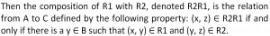Thus, R1R2 = {(1, 2), (3, 2), (3, 4), (5, 4), (7, 2)}

Thus, option (C) is correct.

Please comment below if you find anything wrong in the above post.

QUESTION: 24

Let f be a function from a set A to a set B, g a function from B to C, and h a function from A to C, such that h(a) = g(f(a)) for all a ∈ A. Which of the following statements is always true for all such functions f and g?

Solution:
QUESTION: 25

A function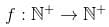. defined on the set of positive integers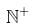. satisfies the following properties :

f(n)=f(n/2)   if n is even

f(n)=f(n+5) if n is odd

Let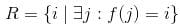be the set of distinct values that f takes. The maximum possible size of R is ______________ .

Solution:

Let us assume: f(1) = x. Then,
f(2) = f(2/2) = f(1) = x
f(3) = f(3+5) = f(8) = f(8/2) = f(4/2) = f(2/1) = f(1) = x
Similarly, f(4) = x f(5) = f(5+5) = f(10/2) = f(5) = y.

So it will have two values. All multiples of 5 will have value y and others will have value x. It will have 2 different values.

QUESTION: 26

A binary relation R on N x N is defined as follows:
(a, b) R (c, d) if a <= c or b <= d.

Consider the following propositions:

P: R is reflexive
Q: R is transitive

Q.
Which one of the following statements is TRUE?

Solution:

THEORY: REFLEXTIVE RELATION:   A relation ‘R’ on a set ‘A’ is said to be reflexive if, (xRx) for every x£A. Ex. If A= {1,2} And R, and P be a relation on AxA, defined as, R= {(2,2),(1,1)} => R is reflexive as it contains all pair of type (xRx). P= {(1,1)} => P is not reflexive relation on A, as it doesn’t contain (2,2).   TRANSITIVE RELATION: A relation ‘R’ on set ‘A’ is said to be transitive if (xRy) and (yRz), then (xRz) for every x,y,z £A. Ex: if A= {1,2} Let R be a relation on AxA, defined as, R= {(1,1),(1,2),(2,1)} => R is transitive.  SOLUTION: Given, (a, b) R (c, d) if a <= c or b <= d i.Check for reflexivity: if an element of set be (a,b) then, (a,b)R(a,b) should hold true. Here, a<=a or b<=b. So, (a,b)R(a,b) holds true. Hence, ‘R’ is reflexive. ii. Check for transitivity:  if elements of set be (2,3),(3,1) and(1,1) Then, (2,3)R(3,1) as 2<=3 And (3,1)R(1,1) as 1<=1 But (2,3)R(1,1) doesn’t hold true as 2>=1 and 3>=1. Hence, R is reflexive but not transitive

QUESTION: 27

For the set N of natural numbers and a binary operation f : N x N → N, an element z ∊ N is called an identity for f, if f (a, z) = a = f(z, a), for all a ∊ N. Which of the following binary operations have an identity?

1. f (x, y) = x + y - 3
2. f (x, y) = max(x, y)
3. f (x, y) = xy
Solution:

I f(x,y) = x+y-3 = x= y+x-3  =>  y=3 Here identity elements is 3 II f(x,y) = max(x,y)=x=max(y,x)  => y=1 Here identity elements is 1 (III f(x,y) =x^y is not same as f(y,x) = y^x. So no identity element.

QUESTION: 28

Given a boolean function f (x1, x2, ..., xn), which of the following equations is NOT true

Solution:

Option A: f (x1, x2, …, xn) = x1’f(x1, x2, …, xn) + x1f(x1, x2, …, xnCase 1: taking x1=0 RHS = 1.f(x1, x2, …, xn) + 0.f(x1, x2, …, xn) RHS =f(x1, x2, …, xn). Case 2: taking x1=1 RHS = 0.f(x1, x2, …, xn) + 1.f(x1, x2, …, xn) RHS =f(x1, x2, …, xn). In both cases RHS=LHS, so, (A) is true Option B: f (x1, x2, …, xn) = x2f(x1, x2, …, xn) + x2’f(x1, x2, …, xnCase 1: taking x2=0 RHS= 0.f(x1, x2, …, xn) + 1.f(x1, x2…,xn) RHS =f(x1, x2, …, xn).Case 2: taking x2=1 RHS = 1.f(x1, x2, …, xn) + 0.f(x1, x2, …, xn) RHS =f(x1, x2, …, xn). In both cases RHS=LHS, so, (B) is true. Option C: f (x1, x2, …, xn) = xn’f(x1, x2, …, 0) + xnf(x1, x2, …,1) Case 1: taking xn=0 RHS= 1.f(x1, x2, …, 0) + 0.f(x1, x2, …, 1) RHS =f(x1, x2, …, 0) Case 2: taking xn=1 RHS = 0.f(x1, x2, …, 0) + 1.f(x1, x2, …, 1) RHS =f(x1, x2, …, 1)In both cases RHS=LHS, so, (C) is true. Option D: f (x1, x2, …, xn) = f(0, x2, …, xn) + f(1, x2, .., xn) Here, no way to equate LHS and RHS so ‘NOT true’. NO term depends on value of ‘x1’.

QUESTION: 29

Consider the following first order logic formula in which R is a binary relation symbol. ∀x∀y (R(x, y)  => R(y, x)) The formula is

Solution:

VxVy R(x,y) => R(y,x) The above given relation is symmetry But, we have both symmetric relastions possible and also possibility of anti symmetric relation  But neither of always holds for all possibilites of sets. => Both are satisfiable but not valid This solution is contributed by Anil Saikrishna Devarasetty. One more solution : We are given a logical formula. So, to be valid it must be a symmetric relation. Hence, Option A is incorrect. Since, it is a logical formula => it is along with it's negation is satisfiable. Hence, option B is correct.

QUESTION: 30

Let P, Q and R be sets let Δ denote the symmetric difference operator defined as PΔQ = (P U Q) - (P ∩ Q). Using Venn diagrams, determine which of the following is/are TRUE? PΔ (Q ∩ R) = (P Δ Q) ∩ (P Δ R) P ∩ (Q ∩ R) = (P ∩ Q) Δ (P Δ R)

Solution: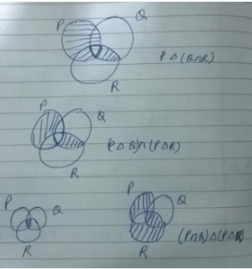Use Code STAYHOME200 and get INR 200 additional OFF Use Coupon Code